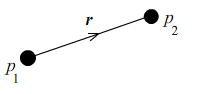#### You may also like### Lunar Leaper

Gravity on the Moon is about 1/6th that on the Earth. A pole-vaulter 2 metres tall can clear a 5 metres pole on the Earth. How high a pole could he clear on the Moon?### Which Twin Is Older?

A simplified account of special relativity and the twins paradox.### Whoosh

A ball whooshes down a slide and hits another ball which flies off the slide horizontally as a projectile. How far does it go?

# Hold Still Please

##### Age 16 to 18 Challenge Level:

Imagine holding a set of point charges at fixed positions in space - you can choose how many, the positions and the sizes of the different charges.

Experiment with different configurations of charges to determine whether you could arrange the charges so that when released simultaneously none of them starts to move.

Could you arrange the charges so that when released simultaneously only $1$ or $2$ of them start to move?

You might use your intuition to explore various possible configurations, but you will need to use Coulomb's law to show that the vector force on a particular particle is zero (see the Notes below) to prove your results.

Imagine that you are allowed to hold fixed one or more of the charges. What are the possibilities now?

Do your configurations seem stable to slight perturbations, in that they won't fly apart when subjected to a slight nudge? How might you create a stable configuration to confine a charged particle?

NOTES AND BACKGROUND

 Coulomb's law states that the electrostatic force ${\bf F_{12}}$ a charged particle $p_1$ of charge $q_1$ feels due to the presence of another charged particle $p_2$ of charge $q_2$ is $${\bf F_{12}} = -\frac{kq_1q_2}{r^2}{\bf \hat{r}}\,,$$ where ${\bf r}$ is the vector displacement of $p_2$ from $p_1$and $k$ is a positive constant, the value of which depends on the electrostatic permittivity of the medium in which the charges reside